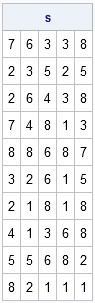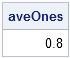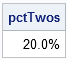Sampling with replacement is a useful technique for simulations and for resampling from data. Over at the SAS/IML Discussion Forum, there was a recent question about how to use SAS/IML software to sample with replacement from a set of events. I have previously blogged about efficient sampling, but this topic is so important that I'm happy to revisit it.

In Chapter 12 of my book, Statistical Programming with SAS/IML Software, I present a complete module for sampling with replacement. The module handles sampling with equal or unequal probabilities. After the book is published, you can download the code for that module.

For now, consider the case of sampling with replacement with equal probability. That is, suppose you have a box with n different items and you want to draw from this box k times. After each draw you replace the item that you took out, so that the probability of drawing a particular item is fixed at 1/n.

After you have drawn one sample of size k, you might want to repeat the procedure by drawing additional samples. This enables you to deal with statistical variation and to estimate probabilities of certain events.

To be concrete, suppose you want to draw a random sample of size k=5 from a set of size n=8. One way to simulate this is to generate 5 random numbers from the uniform distribution. Each of these numbers is in the interval (0,1), so you can map these numbers to the integers 1–8 by multiplying each number by 8 and rounding up the result.

Here is a SAS/IML module for random sampling with replacement with uniform probability:

```proc iml; /** Module for random sampling with replacement and uniform probability. A is row vector with n elements and each sample contains k elements. The result is an nSamples x k matrix. Each row contains one sample. **/ start SampleReplaceUni(A, nSamples, k); results = j(nSamples, k); /** allocate result matrix **/ call randgen(results, "Uniform"); /** fill with random U(0,1) **/ n = ncol(A); /** number of elements **/ results = ceil(n*results); /** convert to integers 1,2,...n **/ return(shape(A[results], nSamples)); /** reshape and return from A **/ finish;```

To test the module:

```call randseed(1234); /** Draw 5 times from {1,2,...,8}. Repeat 10 times. **/ s = SampleReplaceUni(1:8, 10, 5); print s;```When you have multiple samples, you can ask questions such as

• What is the average number of ones that appeared in each sample?
```ones = (s=1)[,+]; aveOnes = ones[:]; print aveOnes;```• In what percentage of the samples did a two appear on the second draw?
```numTwos = (s=2)[+,2]; pctTwos = numTwos / nrow(s); print pctTwos[format=PERCENT7.2];```ShareDistinguished Researcher in Computational Statistics

Rick Wicklin, PhD, is a distinguished researcher in computational statistics at SAS and is a principal developer of SAS/IML software. His areas of expertise include computational statistics, simulation, statistical graphics, and modern methods in statistical data analysis. Rick is author of the books Statistical Programming with SAS/IML Software and Simulating Data with SAS.

1.Hi Rick, I like the blog! I have a question about the RANDGEN routine. Does it utilize the RNG that the RAND data step function uses or the older RNG that's used by RANUNI? If it's the older one, is there any possibility it will be updated? I utilize IML for a lot of Monte Carlo simulations, so I'm very interested. Thanks,

Bob

2.Great question. RANDGEN uses the same Mersenne-Twister random number generator that the RAND function in the DATA step uses. For more on random numbers, see p. 27 of the FREE chapter of my book: http://support.sas.com/publishing/authors/wicklin.html

3.That's great news. I'm lecturing on simulation in SAS in about 10 minutes and I'll tell my students. I suspect they won't be as enthused as I am though.

4.I'm a former professor, so I know the feeling!